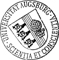## Adaptive Edge Element Approximation of H(curl)-Elliptic Optimal Control Problems with Control Constraints

• A three-dimensional H(curl)-elliptic optimal control problem with distributed control and pointwise constraints on the control is considered. We present a residual-type a posteriori error analysis with respect to a curl-conforming edge element approximation of the optimal control problem. Here, the lowest order edge elements of Nédélec's first family are used for the discretization of the state and the control with respect to an adaptively generated family of simplicial triangulations of the computational domain. In particular, the a posteriori error estimator consists of element and face residuals associated with the state equation and the adjoint state equation. The main results are the reliability of the estimator and its efficiency up to oscillations in terms of the data of the problem. In the last part of the paper, numerical results are included which illustrate the performance of the adaptive approach.

Author: Ronald H. W. HoppeGND, Irwin Yousept urn:nbn:de:bvb:384-opus4-24865 https://opus.bibliothek.uni-augsburg.de/opus4/2486 Preprints des Instituts für Mathematik der Universität Augsburg (2013-19) Preprint English Universität Augsburg 2013/10/11 optimal control of PDEs; H(curl)-elliptic problems; curl-conforming edge elements; residual a posteriori error estimator; reliability and efficiency Elliptische Differentialgleichung; Optimale Kontrolle; A-posteriori-Abschätzung; Fehleranalyse Mathematisch-Naturwissenschaftlich-Technische Fakultät Mathematisch-Naturwissenschaftlich-Technische Fakultät / Institut für Mathematik Mathematisch-Naturwissenschaftlich-Technische Fakultät / Institut für Mathematik / Lehrstuhl für Numerische Mathematik 5 Naturwissenschaften und Mathematik / 51 Mathematik / 510 MathematikDeutsches Urheberrecht mit Print on Demand